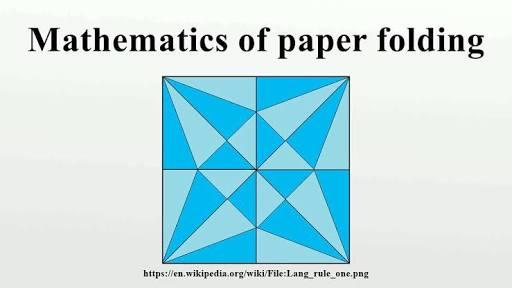# What Do You Know About The Mathematics Of Paper Folding?

Approved & Edited by ProProfs Editorial Team
At ProProfs Quizzes, our dedicated in-house team of experts takes pride in their work. With a sharp eye for detail, they meticulously review each quiz. This ensures that every quiz, taken by over 100 million users, meets our standards of accuracy, clarity, and engagement.
A
Community Contributor
Quizzes Created: 145 | Total Attempts: 37,893
Questions: 10 | Attempts: 145SettingsApparently, this topic is treated under mathematicians as the mathematics of paper folding focuses on using paper folding to solve problems and mathematical equations and demonstrate proofs of certain things. And recently, there have been a great deal of mathematical studies on how to fold pieces of paper. Its history dates back to the 17th century.

• 1.

### What is paper folding aso called?

• A.

Organics

• B.

Origami

• C.

Orifices

• D.

Origins

B. Origami
Explanation
Origami is the correct answer because it is a traditional Japanese art of paper folding. It involves creating intricate and delicate designs by folding paper without the use of scissors or glue. The word "Origami" comes from the Japanese words "ori" meaning folding and "kami" meaning paper. This art form is not only visually appealing but also requires precision and patience. It is widely practiced and admired worldwide for its creativity and beauty.

Rate this question:

• 2.

### Which set of equation do we use the paper fold for?

• A.

Integral equation

• B.

Mathematical equation

• C.

Research analysis

• D.

Infinitesimal equation

B. Mathematical equation
Explanation
The paper fold method is commonly used to solve mathematical equations. By folding a piece of paper and using geometric constructions, it becomes possible to find the solution to certain types of equations. This technique is particularly useful for solving quadratic equations, as it allows for a visual representation of the problem and helps in understanding the roots of the equation. Therefore, the correct answer is Mathematical equation.

Rate this question:

• 3.

### Which of the mathematicians used paper folding to represent geometrical constructions?

• A.

Sundara Rao

• B.

Peter Rudd

• C.

Joshua Sett

• D.

Thomas Johnson

A. Sundara Rao
Explanation
Sundara Rao used paper folding to represent geometrical constructions.

Rate this question:

• 4.

### Which of the following shows the construction of paper folding models?

• A.

Multiple pattern

• B.

Single pattern

• C.

Mid-level surface

• D.

Crease pattern

D. Crease pattern
Explanation
The correct answer is "Crease pattern." A crease pattern is a diagram that shows the folding lines and creases required to construct a paper folding model. It is a visual representation of the steps needed to create the model, indicating where to fold and where to make creases. It serves as a guide for accurately folding the paper and achieving the desired shape or design.

Rate this question:

• 5.

### How do we identify map folding problems?

• A.

When creases are in straight form

• B.

When there are single patterns

• C.

When the different shapes are formed

• D.

When creases are orthogonal

D. When creases are orthogonal
Explanation
When creases are orthogonal, it means that they are at right angles to each other. In the context of map folding, this can be used as a way to identify folding problems. When creases are not orthogonal, it can indicate that the folding is incorrect or that the map is not folded properly. Orthogonal creases are important for creating neat and organized folds, ensuring that the map can be easily unfolded and read without any confusion or distortion.

Rate this question:

• 6.

### How many mathematical rules do we follow to produce flat-foldable patterns?

• A.

2

• B.

3

• C.

4

• D.

5

B. 3
Explanation
To produce flat-foldable patterns, we follow three mathematical rules. These rules ensure that the pattern can be folded without any overlaps or gaps. By following these rules, we can create a flat-foldable pattern that can be easily folded into a three-dimensional shape.

Rate this question:

• 7.

### Which of these is one of the rules used for producing flat-foldable patterns?

• A.

Makeriwk's theorem

• B.

Kovacic's theorem

• C.

Maekawa's theorem

• D.

Silva's theorem

C. Maekawa's theorem
Explanation
Maekawa's theorem is one of the rules used for producing flat-foldable patterns. This theorem states that for a flat-foldable pattern, the sum of the number of edges, number of vertices, and number of faces minus the number of holes must equal 2. This theorem helps in determining whether a pattern can be folded flat without any overlaps or self-intersections.

Rate this question:

• 8.

### In Kawasaki theorem, what is the sum of all odd angles?

• A.

90 degrees

• B.

180 degrees

• C.

270 degrees

• D.

360 degrees

B. 180 degrees
Explanation
The Kawasaki theorem states that in a triangle, the sum of the odd angles (angles opposite odd sides) is equal to 180 degrees. This means that if we add up all the odd angles in a triangle, the total will always be 180 degrees.

Rate this question:

• 9.

### Can a sheet penetrate a fold?

• A.

No

• B.

Yes

• C.

Only when it is evenly divided

• D.

Only when folded into a triangular shape

A. No
Explanation
A sheet cannot penetrate a fold because by definition, a fold involves bending or creasing a material, which creates layers that are stacked on top of each other. Therefore, there is no space for the sheet to pass through the fold.

Rate this question:

• 10.

### Which of the following scientists did NOT work on this model?

• A.

Beloch Margharita

• B.

Humiaki Huzita

• C.

Jacques Justin

• D.

Justine JoaquinBack to top